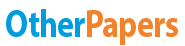# Converting Gravitational Potential Energy to Kinetic Energy

Essay by   •  December 6, 2018  •  Research Paper  •  516 Words (3 Pages)  •  127 Views

## Essay Preview: Converting Gravitational Potential Energy to Kinetic Energy

Report this essay
Page 1 of 3

Do you know how to convert gravitational potential energy to kinetic energy? Conversion is the act of making one thing into another. When you convert these energies then you are making one energy into the other. There are three key things that make the conversion of these energies possible gravitational potential energy, kinetic energy, and force. I hypothesise that, if the potential energy of the object increases then the kinetic energy of the object will also increase.

Gravitational potential energy is energy that does not involve motion, but is instead caused by a change in an object's position or shape. The energy caused by this change is stored within the object itself.For example, an object placed at 100 feet will have more gravitational potential energy than an object placed at 50 feet. This is because the object placed at 100 feet has had a greater change in position. Therefore the object has a greater amount of gravitational potential energy.

Kinetic energy is an energy that does utilize motion. Kinetic energy is caused by the motion or movement that an object does. For example, if one man does 10 jumping jacks and another man does 5 jumping jacks then the man

that did 10 jumping jacks will have a greater amount of kinetic energy.

This is because the man that did 10 jumping jacks did more motion than the man that did 5 jumping jacks. This causes the man that did more jumping jacks to have a greater amount of kinetic energy.

Gravitational potential energy is converted to kinetic energy by force. This happens when an object with potential energy is forced to a place where there is zero gravitational potential energy (usually ground level). This process creates kinetic energy by using force to put the object in motion or to make the object move. For example, if a grape is placed at 100 feet to give it gravitational potential energy and then forced to fall back down by earth's gravitational pull which would give the grape kinetic energy.

In conclusion, the conversion of gravitational potential energy to kinetic energy is one that is by force. Gravitational potential energy is caused by a change in an object's position or shape. While on the other hand, kinetic energy is caused by an object’s motion and/or movement. The conversion of these energies comes when gravitational potential energy is forced to a place where there is no gravitational potential energy therefore creating kinetic energy. That is how gravitational potential energy is converted to kinetic energy.

...

...

Download as:   txt (2.6 Kb)   pdf (37 Kb)   docx (10.3 Kb)
Continue for 2 more pages »
Only available on OtherPapers.com
Citation Generator

(2018, 12). Converting Gravitational Potential Energy to Kinetic Energy. OtherPapers.com. Retrieved 12, 2018, from https://www.otherpapers.com/essay/Converting-Gravitational-Potential-Energy-to-Kinetic-Energy/65151.html

"Converting Gravitational Potential Energy to Kinetic Energy" OtherPapers.com. 12 2018. 2018. 12 2018 <https://www.otherpapers.com/essay/Converting-Gravitational-Potential-Energy-to-Kinetic-Energy/65151.html>.

"Converting Gravitational Potential Energy to Kinetic Energy." OtherPapers.com. OtherPapers.com, 12 2018. Web. 12 2018. <https://www.otherpapers.com/essay/Converting-Gravitational-Potential-Energy-to-Kinetic-Energy/65151.html>.

"Converting Gravitational Potential Energy to Kinetic Energy." OtherPapers.com. 12, 2018. Accessed 12, 2018. https://www.otherpapers.com/essay/Converting-Gravitational-Potential-Energy-to-Kinetic-Energy/65151.html.